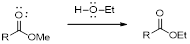Chapter 23, Problem 95GQ

Chapter
Section
Textbook Problem

Draw the structure of glyceryl trilaurate, a fat. Laurie acid (page 941) has the formula C11H23CO2H.(a) Write an equation for the base hydrolysis of glyceryl trilaurate.(b) Write an equation for the reaction that could be used to prepare biodiesel fuel from this fat.

(a)

Interpretation Introduction

Interpretation:

The reaction has to be written for the base hydrolysis of glyceryl trilaurate.

Concept Introduction:

Esters are prepared by the condensation reaction of carboxylic acids and alcohols. One mole of each acid and alcohol undergo condensation reaction and one mole of ester produced.

The reaction of an ester with an alkali base is known as the base hydrolysis of ester. The products obtained after hydrolysis are a sodium salt of carboxylic acid and the alcohol. For example base ydrolysis of ethylacetate gives sodiumacetate and ethyl alcohol.

Explanation

The structure of the glyceryl trilaurate is drawn below.

The equation for hydrolydsis reaction of glyceryl trilaurate is written below.

The base hydrolysis of glyceryl-trilaurate gives one mole of glycerol and three moles of sodium salt of lauric acid...

(b)

Interpretation Introduction

Interpretation:

The reaction equation has to be written which can be used to prepare bio-deisel from this fat.

Concept Introduction:

Transesterification: Transesterification reaction is an esterification reaction of ester react with excess of alcohol in the presence of either acid or base catalyst to form a new ester. The formation of one type of ester can be transformed in to other form of esters is called esterification when reaction moves forward when we use excess of an alcohol.

The mechanism of the transesterification sequence of arrow pushing pattern is given.Still sussing out bartleby?

Check out a sample textbook solution.

See a sample solution

The Solution to Your Study Problems

Bartleby provides explanations to thousands of textbook problems written by our experts, many with advanced degrees!

Get Started

Find more solutions based on key concepts